Home > CALC > Chapter 8 > Lesson 8.4.1 > Problem8-142

8-142.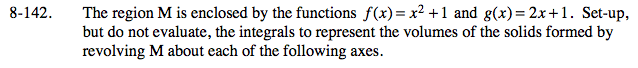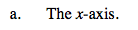Sketch the axis of rotation (flag pole) then use washers.

$V=\pi \int_{x=a}^{x=b}(R)^{2}-(r)^{2}dx$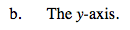Use shells.

$V=2\pi \int_{x=a}^{x=b}xf(x)dx$

Note the x represents the length of the radius, it's part of the base = 2πr equation. And f(x) represents the height of each shell.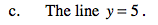Refer to the hint in part (a).

Recall that R and r represent radii of an outer and inner solid (the 'inner' solid is the hole). Make R the function with the longer radii and r the function with the shorter radii. Note: It is possible that R is below r on the graph. Once rotation happens, this will switch.

Also note that both radii have different lengths than f(x) and g(x), since the axis of rotation is 5 units above the x-axis. Adjust the integral in part (a) accordingly:

$V=\pi \int_{x=a}^{x=b}(k-R)^{2}-(k-r)^{2}dx$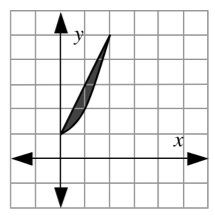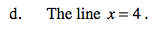As in part (b), to avoid using horizontal rectangles (and rewriting the integrandin terms of y), you can use shells.

The setup should look exactly like the setup in part (b), with one exception: The radii are no longer the same values as the bounds. They will be either longer or shorter than the bounds. So shift the radii k units to the left or right:

$V=2\pi \int_{x=a}^{x=b}(x-k)f(x)dx$